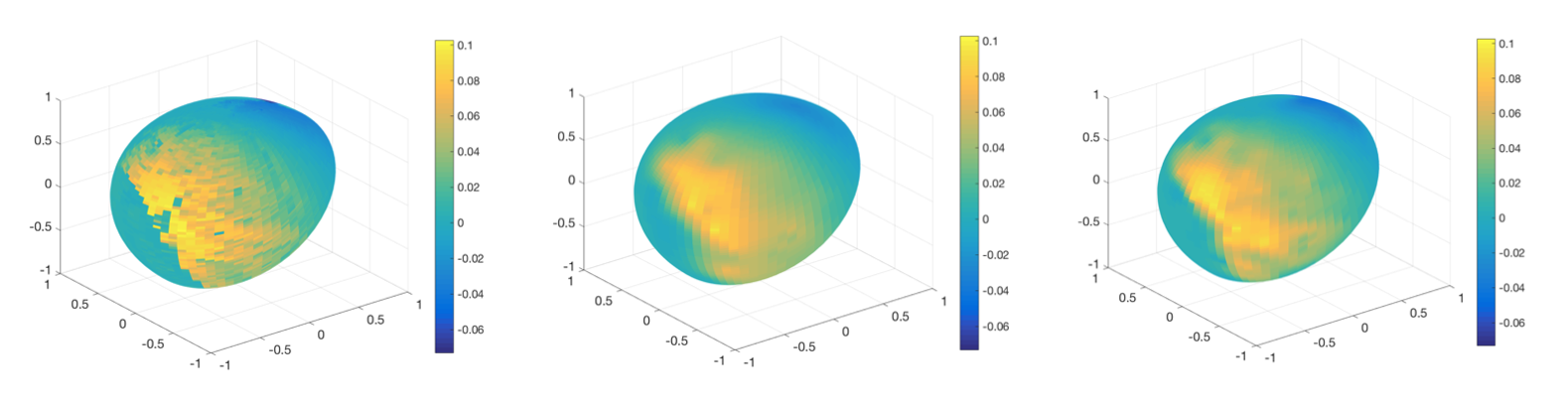# Transfer Learning for Numerical Integration

## The problem

Estimating integrals or expectations through simulation is one of the key challenges in computational statistics and machine learning. Indeed, many quantities of interest including posterior moments, model evidences, tail probabilities and marginal likelihoods take the form of intractable integrals, and we hence need algorithms to obtain accurate estimates. One of the key challenges is the cost (either computational or financial) of obtaining integrand evaluations. When working with complex models, this cost can be very large, which limits the number of integrand evaluations available and can lead to poor accuracy of the resulting algorithms. In order to obtain highly accurate estimates of these integrals, it is therefore necessary to make use of any mathematical structure or side-information available.

There are of course many different types of side-information you could attempt to encode in algorithms, but one key element which is often neglected is that we rarely have to compute a single isolated integral. Indeed, many practical problems will require you to compute several related integrals either simultaneously or sequentially. For example, in Bayesian statistics you usually have to compute some posterior expectation of interest, but you will also often want to calculate similar posterior expectations if you collect more data or want to try out different priors. Similarly, when working with mathematical models of physical or biological phenomenon, you will often have access to models of varying accuracy and may want to calculate integrals for each of these. In cases where the various integration problems are similar enough, it may be worth designing algorithms which can share integrand evaluations across tasks in order to improve accuracy.

## Contributions to this field

My main contribution to answering this problem has been a series of papers which propose to use transfer learning for computing integrals. Transfer learning is a general paradigm in machine learning where algorithms are designed to transfer information across related tasks, and hence improve accuracy. There is a wide range of applications of transfer learning including for regression and classification, but there is surprisingly little in the context of numerical integration.

(see the related Bayesian numerical methods page for more details)

• Xi, X., Briol, F-X. & Girolami, M. (2018). Bayesian quadrature for multiple related integrals. International Conference on Machine Learning, PMLR 80:5369-5378. (Conference) (Preprint)

• Sun, Z., Barp, A. & Briol, F-X. (2021). Vector-valued control variates.## Funding

Funding for this work was generously provided by Amazon through an Amazon Research Award for a project entitled “Transfer Learning for Numerical Integration in Expensive Machine Learning Systems”. See the following Amazon announcement and UCL press release for more details.